# • Buffer conceptual and calculation: • A chemist needs a pH 3.33 buffer. Select the best...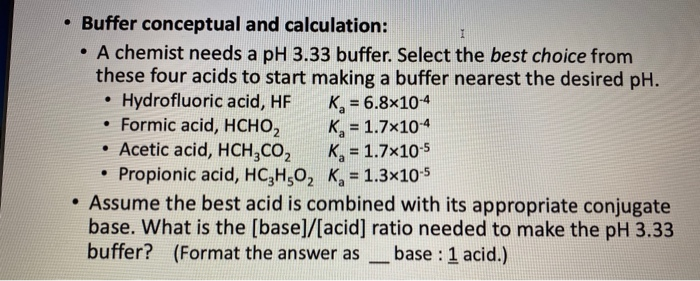• Buffer conceptual and calculation: • A chemist needs a pH 3.33 buffer. Select the best choice from these four acids to start making a buffer nearest the desired pH. • Hydrofluoric acid, HF K = 6.8x10-4 • Formic acid, HCHO, Kg = 1.7x10-4 • Acetic acid, HCH,CO, K= 1.7x10-5 • Propionic acid, HC2H50, Ks = 1.3x10-5 Assume the best acid is combined with its appropriate conjugate base. What is the [base]/[acid) ratio needed to make the pH 3.33 buffer? (Format the answer as _ base : 1 acid.)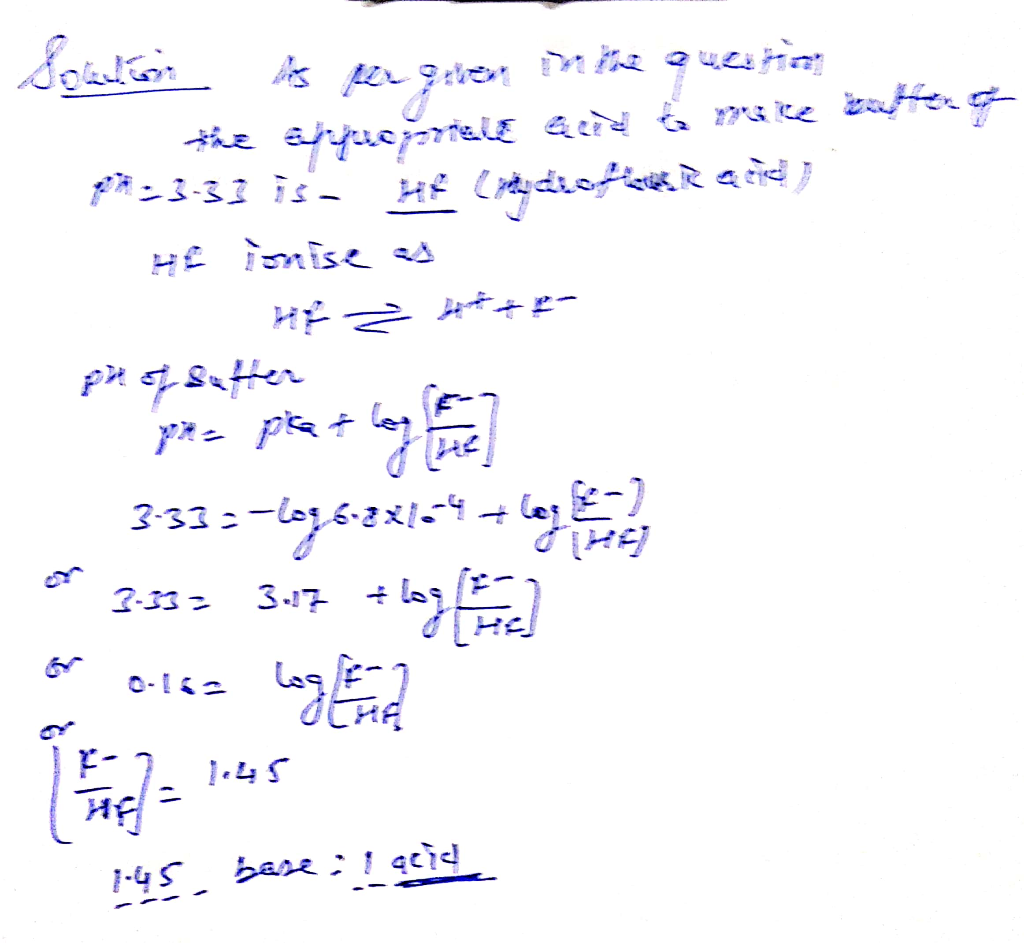#### Earn Coin

Coins can be redeemed for fabulous gifts.

Similar Homework Help Questions
• ### I’m confused on how to do the question. Please help Lecture 15 At-home Practice Buffer conceptual...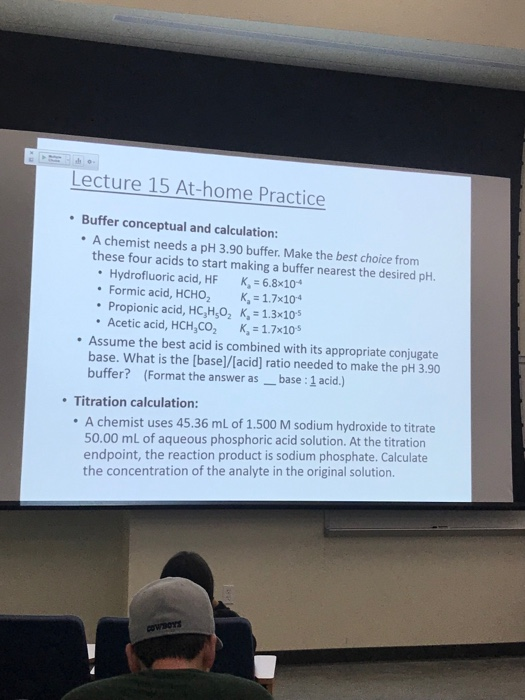I’m confused on how to do the question. Please help Lecture 15 At-home Practice Buffer conceptual and calculation: A chemist needs a pH 3.90 buffer. Make the best choice from these four acids to start making a buffer nearest the desired pH. * Hydrofluoric acid, HF Ka-6.8x104 . Formic acid, НСНО, K,-1.7x10-4 Propionic acid, HC,HO2 K, 1.3x105 Acetic acid, HCH,CO2 K, 1.7x10 Assume the best acid is combined with its appropriate conjugate base. What is the [base]/[acid] ratio needed to...

• ### Determine which weak acid is the best option to make a buffer at the specified pH...

Determine which weak acid is the best option to make a buffer at the specified pH of 3.00. ***formic acid, ?a=1.77×10−4, 2.00 M propionic acid, ?a=1.34×10−5, 3.00 M phosphoric acid, ?a=7.52×10−3, 1.00 M acetic acid, ?a=1.75×10−5, 5.00 M Determine which conjugate base is the best option to make a buffer at the specified pH. sodium dihydrogen phosphate monohydrate, NaH2PO4⋅H2O ***sodium formate, HCOONa sodium acetate trihydrate, CH3COONa⋅3H2O sodium propionate, CH3CH2COONa The final volume of buffer solution must be 100.00 mL and...

• ### 1) You are to make a buffer with a pH of 4.40. Below are the acids...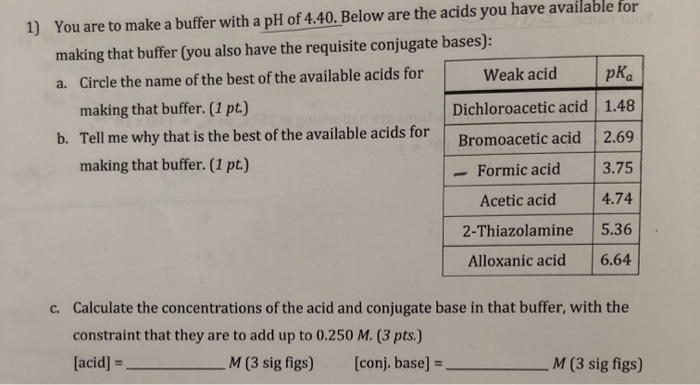1) You are to make a buffer with a pH of 4.40. Below are the acids you have available for making that buffer (you also have the requisite conjugate bases): a. Circle the name of the best of the available acids for Weak acid |pka making that buffer. (1 pt.) Dichloroacetic acid 1.48 b. Tell me why that is the best of the available acids for Bromoacetic acid 2.69 making that buffer. (1 pt.) - Formic acid 3.75 Acetic acid...

• ### What is the pH of a buffer solution containing 0.13 M HF and 5.0×10−2 M NaF?...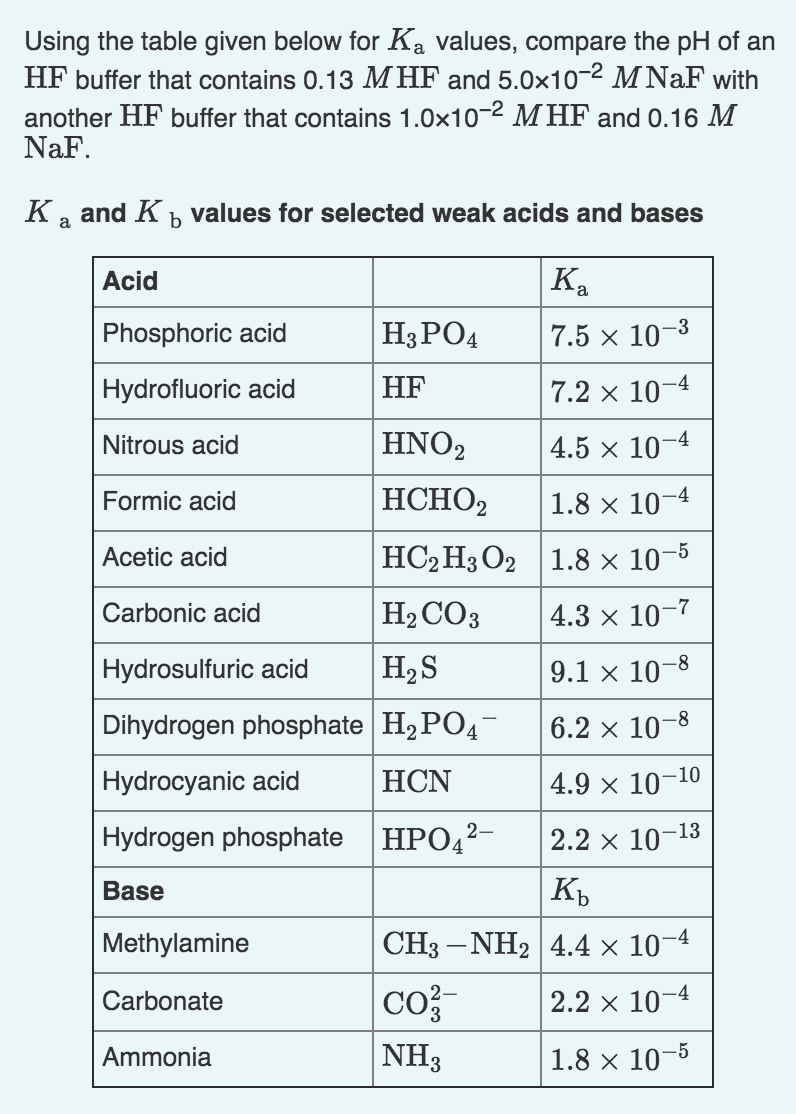What is the pH of a buffer solution containing 0.13 M HF and 5.0×10−2 M NaF? What is the pH of a buffer solution containing 1.0×10−2 M HF and 0.16 M NaF? Using the table given below for Ka values, compare the pH of an HF buffer that contains 0.13 MHF and 5.0x10-2 M NaF with another HF buffer that contains 1.0x10-2 MHF and 0.16 M NaF. Ka and K b values for selected weak acids and bases HF Acid...

• ### A student must make a buffer solution with a pH of 1.00, Determine which weak acid...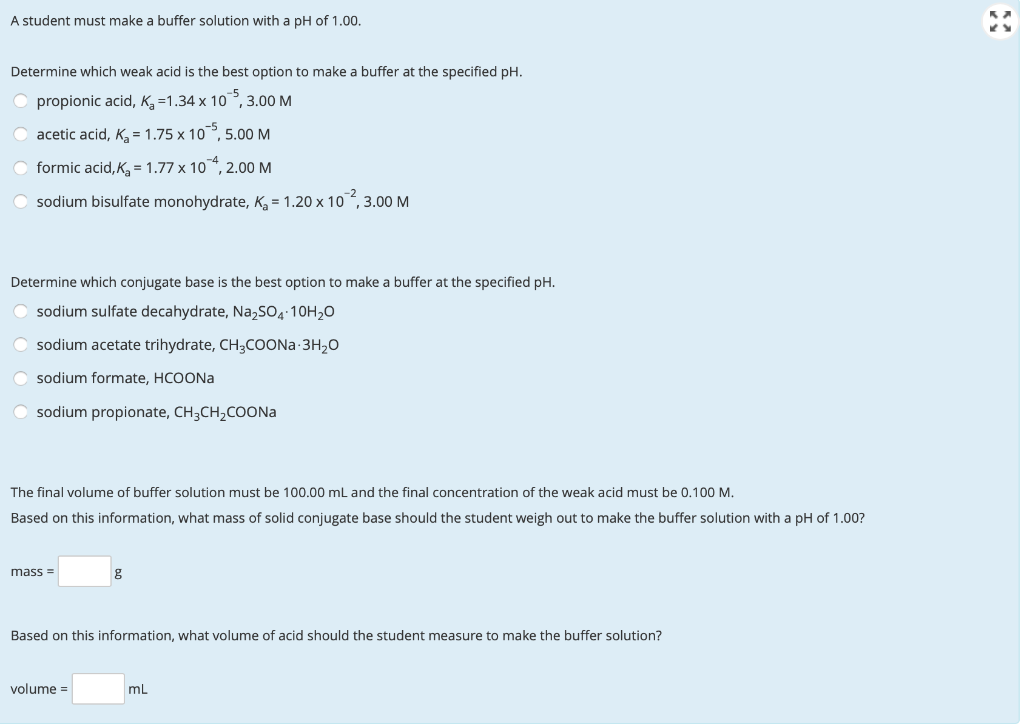A student must make a buffer solution with a pH of 1.00, Determine which weak acid is the best option to make a buffer at the specified pH. propionic acid, Kg =1.34 x 10,3.00 M acetic acid, Kg = 1.75 x 105,5.00 M formic acid, Kg = 1.77 x 104, 2.00 M sodium bisulfate monohydrate, K = 1.20 x 10,3.00 M Determine which conjugate base is the best option to make a buffer at the specified pH. sodium sulfate decahydrate,...

• ### A student must make a buffer solution with a pH of 1.00. Determine which weak acid...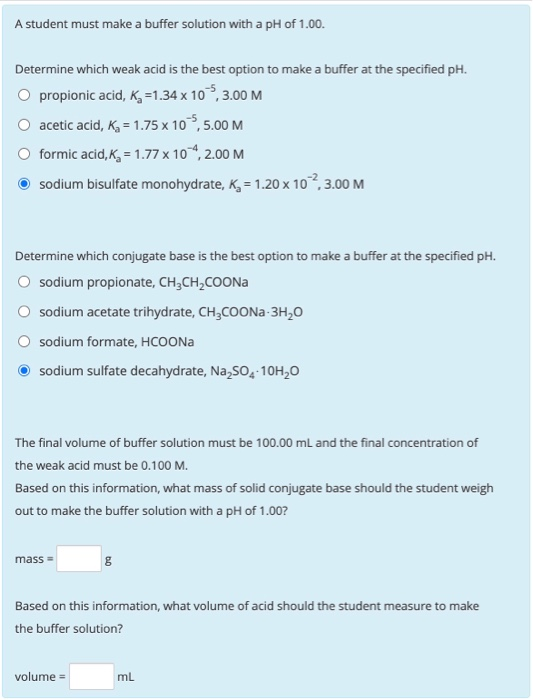A student must make a buffer solution with a pH of 1.00. Determine which weak acid is the best option to make a buffer at the specified pH. O propionic acid, K =1.34 x 10 5,3.00 M acetic acid, Ka = 1.75 x 10,5.00 M O formic acid, Kg = 1.77 x 104,2.00 M sodium bisulfate monohydrate, K = 1.20 x 102,3.00 M Determine which conjugate base is the best option to make a buffer at the specified pH. sodium...

• ### A student must make a buffer solution with a pH of 2.00. Determine which weak acid...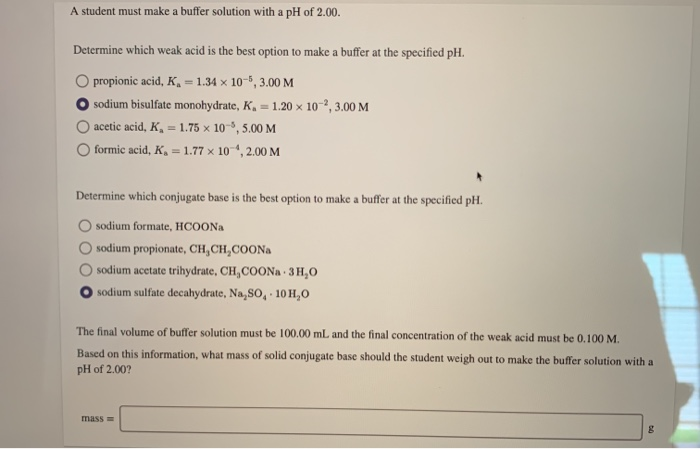A student must make a buffer solution with a pH of 2.00. Determine which weak acid is the best option to make a buffer at the specified pH. O propionic acid, K = 1.34 x 10-6, 3.00 M O sodium bisulfate monohydrate, K = 1.20 x 10,3.00 M acetic acid, K, = 1.75 x 10-6, 5.00 M formic acid, K, = 1.77 x 10 , 2.00 M Determine which conjugate base is the best option to make a buffer at...

• ### Analytical i. As the ionic strength, μ +0, activity coefficient, γ activity - The pH of...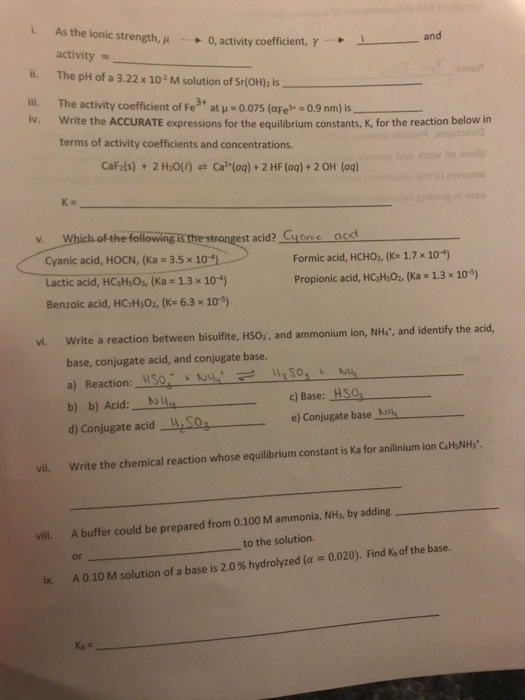Analytical i. As the ionic strength, μ +0, activity coefficient, γ activity - The pH of a 3.22 x 102 M solution of Sr(OH)2 is ii. The activity coefficient of Fe3t at μ = 0.075 (aFe"-o9 nm) is Write the ACCURATE expressions for the equilibrium constants, K, for the reaction below in terms of activity coefficients and concentrations. v. CaF2(s) + 2 H2O() Ca2"(aq) + 2 HF (aq) + 2 OH (aq) v. Which of the following is the strangest...

• ### A student must make a buffer solution with a pH of 1.50. Determine which weak acid...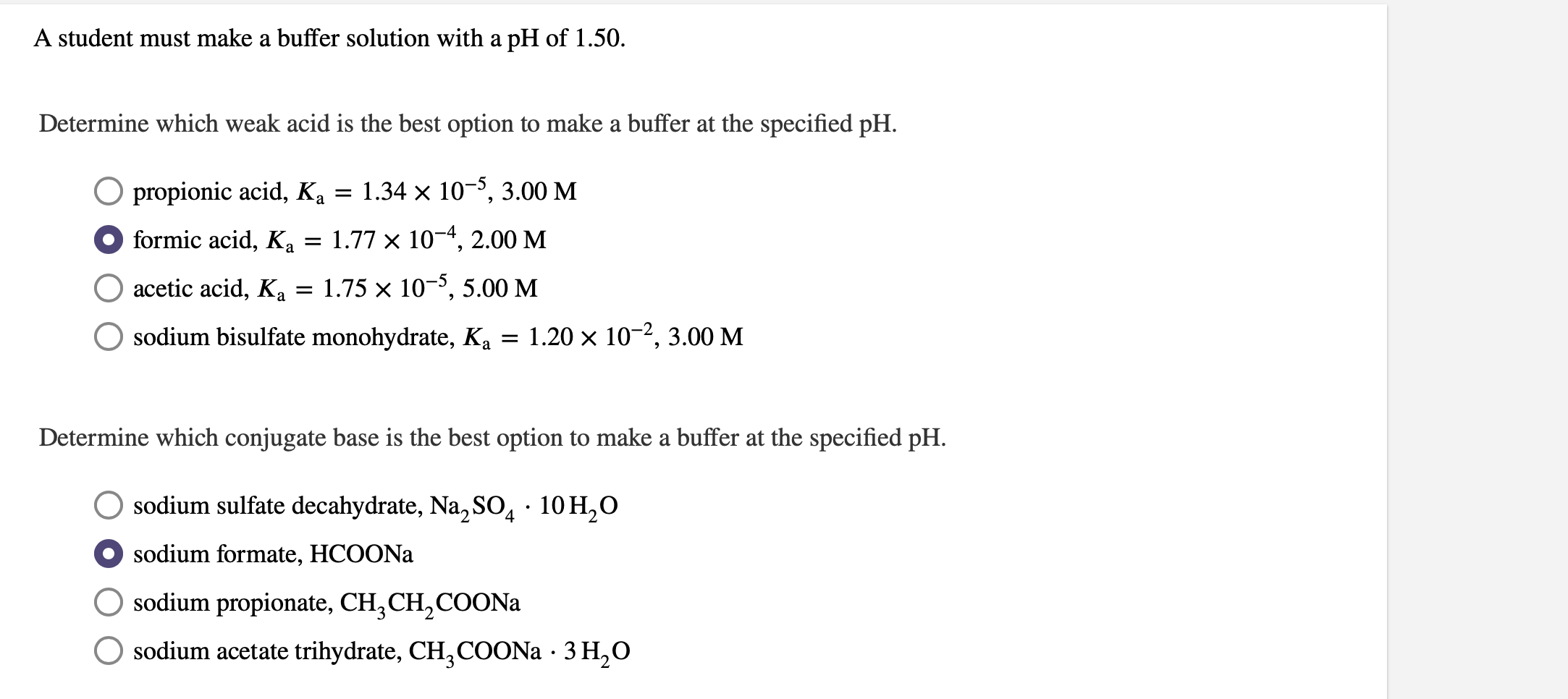A student must make a buffer solution with a pH of 1.50. Determine which weak acid is the best option to make a buffer at the specified pH. O propionic acid, Ka = 1.34 x 10-5, 3.00 M formic acid, Ka = 1.77 x 10-4, 2.00 M O acetic acid, Ka = 1.75 x 10-5,5.00 M O sodium bisulfate monohydrate, Ka = 1.20 x 10-2, 3.00 M Determine which conjugate base is the best option to make a buffer at...

• ### 7. You need to make a buffer to keep a solution at a pH of 2.50....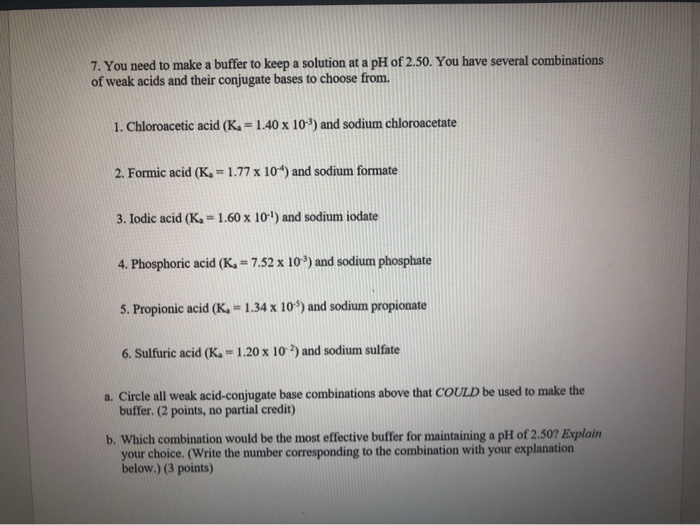7. You need to make a buffer to keep a solution at a pH of 2.50. You have several combinations of weak acids and their conjugate bases to choose from. 1. Chloroacetic acid (K. = 1.40 x 10") and sodium chloroacetate 2. Formic acid (K= 1.77 x 104) and sodium formate 3. Iodic acid (K-1.60 x 10-') and sodium iodate 4. Phosphoric acid (K, = 7.52 x 10%) and sodium phosphate 5. Propionic acid (K, - 1.34 x 10 %)...

Free Homework App